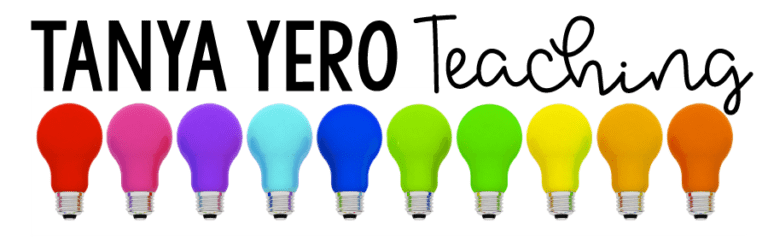# 3rd Grade Word Problems Morning Work | YEARLONG Math Spiral Review Test Prep

\$13.00

Total Pages: 105
File Size: 69 MB

## Description

Visual representation is important to students. Get your students excited about math by Using Snapshots of Math Word Problems!

This purchase contains 100 word problems for all the 3rd grade math standards. Each word problem is applicable for young learners and includes a photo for visual representation. Each question is on a PowerPoint slide for easy use and can be used during a whole group lesson, to introduce a skill, or extra practice.

Standards & Topics Covered

Number and Operation in Base Ten
➥ 3.NB.1 – Place value concepts
➥ 3.NBT.2 – Adding and subtracting whole numbers
➥ 3.NBT.3 – Multiplying numbers

Operations & Algebraic Thinking
➥ 3.OA.1 – Interpreting products of whole numbers
➥ 3.OA.2 – Interpreting quotients of whole numbers
➥ 3.OA.3 – Use multiplication and division to solve word problems
➥ 3.OA.4 – Determining unknown numbers in a multiplication or division equation
➥ 3.OA.5 – Apply properties of operations to multiply and divide
➥ 3.OA.6 – Understand division as an unknown-factor problem.
➥ 3.OA.7 – Fluently multiply and divide within 100
➥ 3.OA.8 – Solve two-step word problems using the four operations.
➥ 3.OA.9 – Understanding patterns in multiplication/addition

Number and Operation – Fractions
➥ 3.NF.1 – Understanding fractions
➥ 3.NF.2 – Understanding fractions on number lines
➥ 3.NF.3 – Equivalent fractions and comparing fractions

Measurement and Data
➥ 3.MD.1 – Understanding time
➥ 3.MD.2 – measuring and understanding liquid volume and mass
➥ 3.MD.3 – Picture graphs
➥ 3.MD.4 – Measuring length and using line plots
➥ 3.MD.5 – Understanding area
➥ 3.MD.6 – Measuring area
➥ 3.MD.7 – Relate area to the operations of multiplication and addition.
➥ 3.MD.8 – Word problems with area and perimeter

Geometry
➥ 3.G.1 – Understanding and examining shapes
➥ 3.G.2 – Partition shapes into parts with equal areas.